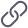# 数学之美第二章读后感 自然语言处理从规则到统计的演变 及 个人新学期代码规范

、  这是一份算法课的作业，要求是阅读吴军博士所著的《数学之美》其中一章并写下读后感。

The pen is in the box.

The box is in the pen.

## 个人代码规范

state-of-the-art-shitcodehttps://github.com/trekhleb/state-of-the-art-shitcode

### 1.变量命名

输出一个值：value 一个临时变量：temp.

### 2.代码规整

import java.math.BigDecimal;
import java.util.Scanner;
public class Exercise2_13 {
public static void main(String[] args){
double amount;
double value;
Scanner input = new Scanner(System.in);
System.out.print("Enter the monthly saving amount:");
amount= input.nextDouble();
value= (double) (amount*(1+0.00417));
for(int i=0;i<5;i++)
{
value= (double) ((amount+value)*(1+0.00417));
}
BigDecimal d = new BigDecimal(value);
value = d.setScale(2,BigDecimal.ROUND_DOWN).doubleValue();
System.out.println("After the sixth month, the account value is $"+ String.format("%.2f",value)); } } 这是java基础第11版的2-13题，题目要求输入一个账户每月的进账金额，并给定一个利率，要你输出6个月后账户的余额，可以看到变量都很清楚。代码需要规整分布，而不是全部挤在一行或者花括号分离。 ### 3.写注释 上文中的代码基本上初学者都看得懂，只不过使用的BigDecimal d的作用可能需要标明。 import java.math.BigDecimal;//直接保留小数点后位数所使用的包 import java.util.Scanner; public class Exercise2_13 { public static void main(String[] args){ double amount; double value; Scanner input = new Scanner(System.in); System.out.print("Enter the monthly saving amount:"); amount= input.nextDouble(); value= (double) (amount*(1+0.00417)); for(int i=0;i<5;i++) { value= (double) ((amount+value)*(1+0.00417)); } BigDecimal d = new BigDecimal(value);//使用BigDecimal d承接我们要输出的账户余额 value = d.setScale(2,BigDecimal.ROUND_DOWN).doubleValue();//直接保留两位小数 System.out.println("After the sixth month, the account value is$"+ String.format("%.2f",value));
}
}

### 4.不要保留没有用的代码，以及无法执行的语句！

08-03479
10-0616
01-13345
11-06223
12-24634
09-04206
01-09315
04-08133
08-16221
09-20629
12-01463
09-13285

### “相关推荐”对你有帮助么？

•非常没帮助
•没帮助
•一般
•有帮助
•非常有帮助被折叠的  条评论 为什么被折叠?到【灌水乐园】发言¥1 ¥2 ¥4 ¥6 ¥10 ¥20获取中扫码支付点击重新获取扫码支付1.余额是钱包充值的虚拟货币，按照1:1的比例进行支付金额的抵扣。
2.余额无法直接购买下载，可以购买VIP、付费专栏及课程。余额充值